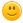# What is 90\$ -60\$.

257 views
asked Mar 24, 2013 in Math

What is 123-56-4 and what is 44+78.Thank you!

answered Apr 24 by (12,330 points)
1. \$30

2. 63

3. 122
+1 vote
answered Feb 17, 2020 by (26,880 points)
IDK
commented Feb 17, 2020 by (211,730 points)
Lol
+1 vote
answered Jul 5, 2017 by (85,770 points)
2 lazy 2 get a calculator but I know that \$90-\$60=\$30
answered Jul 21, 2014 by (425,020 points)
44

+78

_____

122

So do 40+70=110

then 4+8=12

so it's 122

then  \$90-\$60=

thats 30 dollars

123-56-4

123

-56

-4

____

123-60= 63
+1 vote
answered Mar 27, 2013 by (32,950 points)
123-56-4=63 and 44+78=122 hope this helps!
commented Jul 21, 2014 by (425,020 points)
it's a little problem but \$90-\$30
+1 vote
answered Mar 24, 2013 by (18,130 points)
\$90 - &60 is \$30.

123 - 5 - 4 is 114.

44 + 78 is 122.

Hope it helps.

-CraftyGirlJuJu
commented Mar 24, 2013 by (32,370 points)
Sorry, CraftyGirlJuJu, you should check your math for the last two problems.  44 +78 is 122. But, it's okay, you were only one off!
commented Mar 25, 2013 by (18,130 points)
Thanks, I guess in checking it on a calculator wasn't so great. I had 122 and then, I checked with a calculator and I got 121! Thanks so much! I'll edit that.
commented Jan 3, 2014 by (390 points)
She stated that 44 + 78 is 122 smile828
I can buy you a calculator for you if you want!